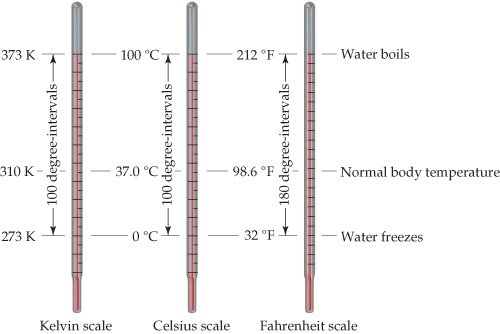# Problem: Comparison of the Kelvin, Celsius, and Fahrenheit temperature scales.True or false: The "size" of a degree on the Celsius scale is the same as the "size" of a degree on the Kelvin scale.

###### FREE Expert Solution

size of a degree on the Celsius scale = size of a degree on the Fahrenheit scale

95% (11 ratings)###### Problem DetailsComparison of the Kelvin, Celsius, and Fahrenheit temperature scales.

True or false: The "size" of a degree on the Celsius scale is the same as the "size" of a degree on the Kelvin scale.

Frequently Asked Questions

What scientific concept do you need to know in order to solve this problem?

Our tutors have indicated that to solve this problem you will need to apply the Temperature concept. You can view video lessons to learn Temperature. Or if you need more Temperature practice, you can also practice Temperature practice problems.